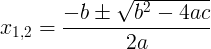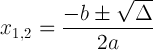Enter the quadratic equation coefficients a, b, c and press the Calculate button:

 Enter a: Enter b: Enter c: The quadratic equation: x2 + x + = 0 Discriminat: Δ  = Quadratic formula: x1,2= First root: x1   = Second root: x2 =

The quadratic equation is given by:

ax2 + bx + c = 0

The quadratic formula is given by:The discriminat is:

Δ = b2 - 4ac

The quadratic formula with discriminat is: